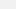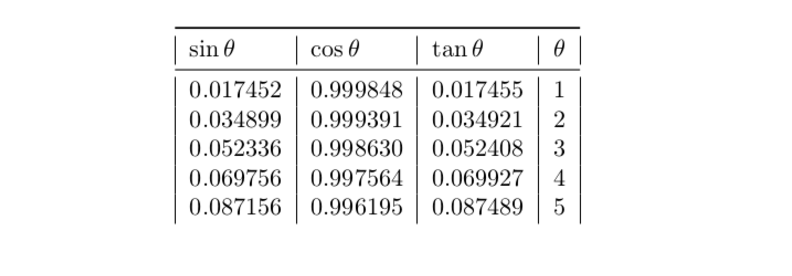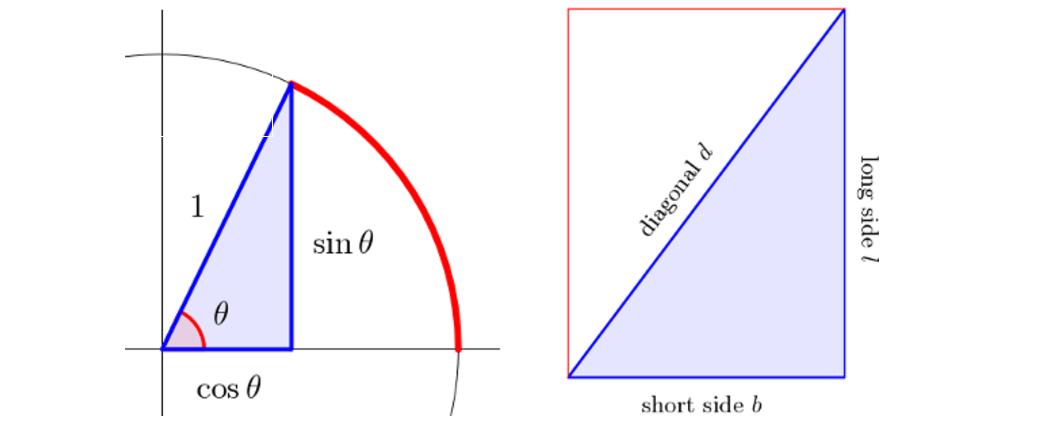# Did the Babylonians Beat the Greeks to Trigonometry By 1000 Years?

## An ancient clay tablet may be the oldest and most accurate trigonometry table ever discovered.

|
Aug 26 2017, 2:00pmImage: Wikimedia Commons

A little over 2,000 years ago, the Greek mathematician Hipparchus of Nicaea created a table that formalized a branch of mathematics called trigonometry. As the discipline devoted to studying the relationships between a triangle's angles and sides, trigonometry had been used for hundreds of years by the Egyptians and Babylonians to design pyramids and do rudimentary astronomy. But these pre-Greek societies lacked the concept of an angle measure, so they were unable to study trigonometry proper until Hipparchus' breakthrough.

At least that's the story anyone who has ever had to suffer through a trig class in high school was told. But according to new research from the University of New South Wales, the Babylonians appear to have formalized the study of angles in trigonometry a full 1,000 years before the Greeks.

This discovery is based on a new interpretation of Plimpton 322, a 3,700-year-old clay tablet discovered in the early 20th century in Iraq. In the 1940s, it was shown that the cuneiform numbers on the tablet corresponded to the Pythagorean Theorem, which states that the square of a right triangle's hypotenuse (the long side) is equal to the squared lengths of its other two sides.

But for 70 years, no one could figure out what the tablet had been used for because the values for the sines and cosines (the ratios between the different sides of a right triangle) were missing. Without further evidence, many scholars concluded that the tablet was not a trigonometric table used for determining the ratios of a triangle's sides, but an ancient school text.

But according to Daniel Mansfield, a mathematician at New South Wales and the lead author of the new paper, seeing the tablet as a trigonometric table just required a shifting of the frame of reference.

On Mansfield's new interpretation, the Plimpton tablet explored trigonometry through the ratios of the sides of a right triangle using the Babylonian base 60 form of mathematics, rather than using angles and the base 10 system we are familiar with today. For our purposes, the main differences between the two systems are that base 10 only allows four factors (1, 2, 5, and 10), whereas base 60 allows for 12 factors (60, 30, 20, 15, 12, 10, 6, 5, 4, 3, 2, and 1).

The practical implications of this is that a culture using a base 60 system can get far more accurate values when doing the division to calculate the ratios for the sides of a triangle than the decimal approximations resulting from the same calculations in base 10.

To see why this is the case, first consider the Greek trigonometry we are familiar with today. We learn that the shape of a right triangle depends on the size of the other two angles, each of which can be calculated by considering the ratios of the triangle's sides. The trigonometric functions used to describe these ratios are called sine, cosine, and tangent, which represent the ratio of the opposite side to the hypotenuse, adjacent side to the hypotenuse, and the opposite side to the adjacent side, respectively.

What this means is that if I know the value of one of the two non-right angles of a triangle, I can easily calculate the ratio of its side as cosine, sine or tangent. Conversely, if I know the value of one of these trigonometric functions, I can easily determine the value of the angle.The value of the angle for a right triangle is shown in the far right column. The ratios of the various sides are depicted in each of the columns for the trigonometric functions. Image: Daniel Mansfield

As you've probably noticed in the above table, the values of the trigonometric functions are approximate—most of the ratios in the table will have values that extend far beyond six decimal places. This is a consequence of the angle in the equation being related to a circle, which is divided into 360 degrees. The upshot of this is that any conceivable triangle can be described in a table, but the price to pay is unwieldy decimals a return values.

The Babylonians, on the other hand, related their triangles to a rectangle, rather than a circle. So instead of using the trigonometric functions we're familiar with today, they used the ratios of the short to long side and the diagonal side to the long side of the triangle to generate a wide variety of right angle triangles. As Manfield points out, this method is both more exact and simpler than the trigonometric system invented by the Greeks 1,000 years later.How we do trigonometry today, versus how the Babylonians did trigonometry. Image: Daniel Mansfield/ The Conversation

"This is a whole different way of looking at trigonometry," Mansfield told Science. "We prefer sines and cosines … but we have to really get outside our own culture to see from their perspective to be able to understand it."

Looked at this way, the 15 lines on the Plimpton tablet represent various ratios for the sides of a right triangle. Starting from the top line and going to the bottom line, the tablet lists series of three numbers that correspond to the Pythagorean theorem and result in 15 exactly calculated right triangles.

Mansfield argues argues that the tablet could have found a number of practical uses in Babylonian society, ranging from surveying fields to an architectural tool used to create palaces and step pyramids.

Mansfield's interpretation of the Plimpton tablet is considered controversial and far from total proof that the tablet is indeed a trigonometric table. Further evidence that the Babylonians put the trigonometric principles supposedly contained in the table to use in their society or another tablet depicting the same trigonometric table would be needed to put Mansfield's interpretation beyond a doubt.